# Vision & Language Pretraining 总结

## UNITER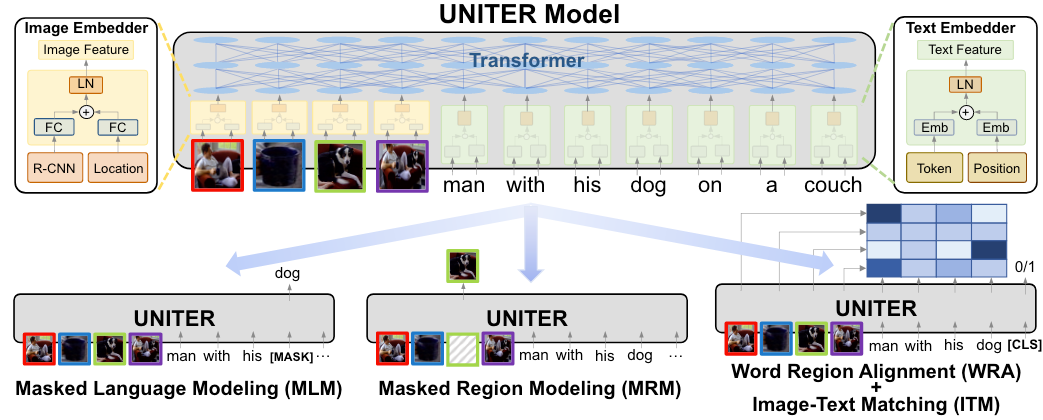UNITER是典型的单塔模型, 直接把Region Feature也变成Token Level Feature和Token Emebdding(Text)一并交给Transformer完成各类预训练任务.

UNITER有四个预训练任务:

• MLM - Masked Language Modeing: 和BERT相同, 对文本打[MASK] 预测原来的Token.
• MRM - Masked Region Modeling: 把Region Vector变成全0, 但是由于Visual Feature是连续的, 没法像NLP的Token一样被多分类预测出来, 所以作者提出了三个MRM的变体:
• MRC - Masked Region Classification: 用Linear预测ROI Feature的Semantic Class. 这里没有Ground Truth Label, 直接用Fast RCNN预测的物体类别当做Ground Truth Label.
• MRC-kl - Masked Region Classification with KL-Divergence: 由于MRC中的Label过于硬了, 所以MRC-kl用Soft Label当做Ground Truth.
• ITM - Image-Text Matching: 采样若干负样本, 用[CLS]接一个Linear来判断图文是否匹配.
• WRA - Word Region Aligment: 粒度比ITM更细, 对齐每个Text Token和Region. 用最优传输来计算二者之间的相似性.

## Oscar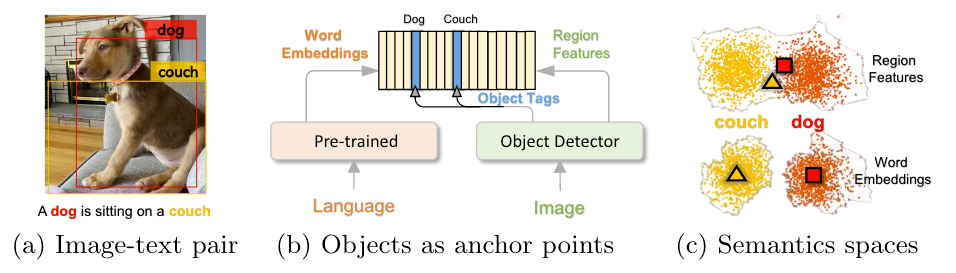Region Feature可能不能很好的区分开不同的物体, 例如图中的dog和couch实际上很大部分重叠到了一起, 但是在Word Embedding Space上二者是分开的.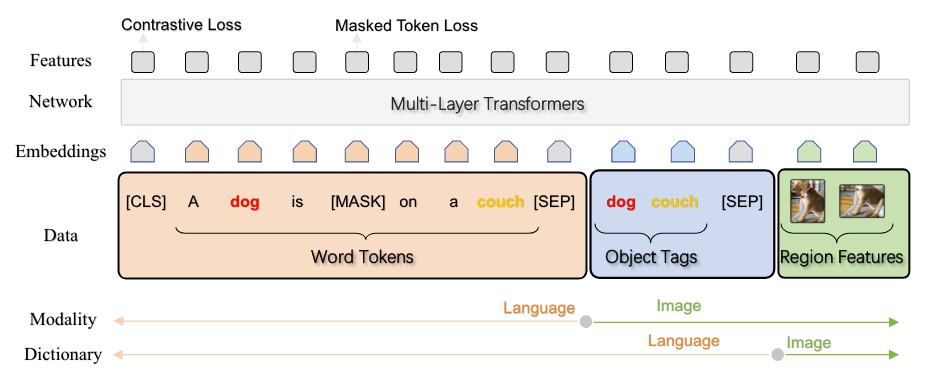Tag本身具备Language的语义, 但实际上描述的是Visual信息, 所以Tag打通了V&L的桥梁.

• Dictionary View: Tag和Text都属于同一个Word Dictionary下, 所以沿用BERT的MLM任务, 要求模型用剩余的Tag或Text, Region Feature来恢复.
• Modality View: Tag和Region Feature同属Visual Signal, 作者令训练阶段有50%的概率替换Tag为负样本, 用[CLS]来预测Tag是否被替换.

## CLIP

CLIP的模型和训练方法非常简单, A picture is worth a thousand words: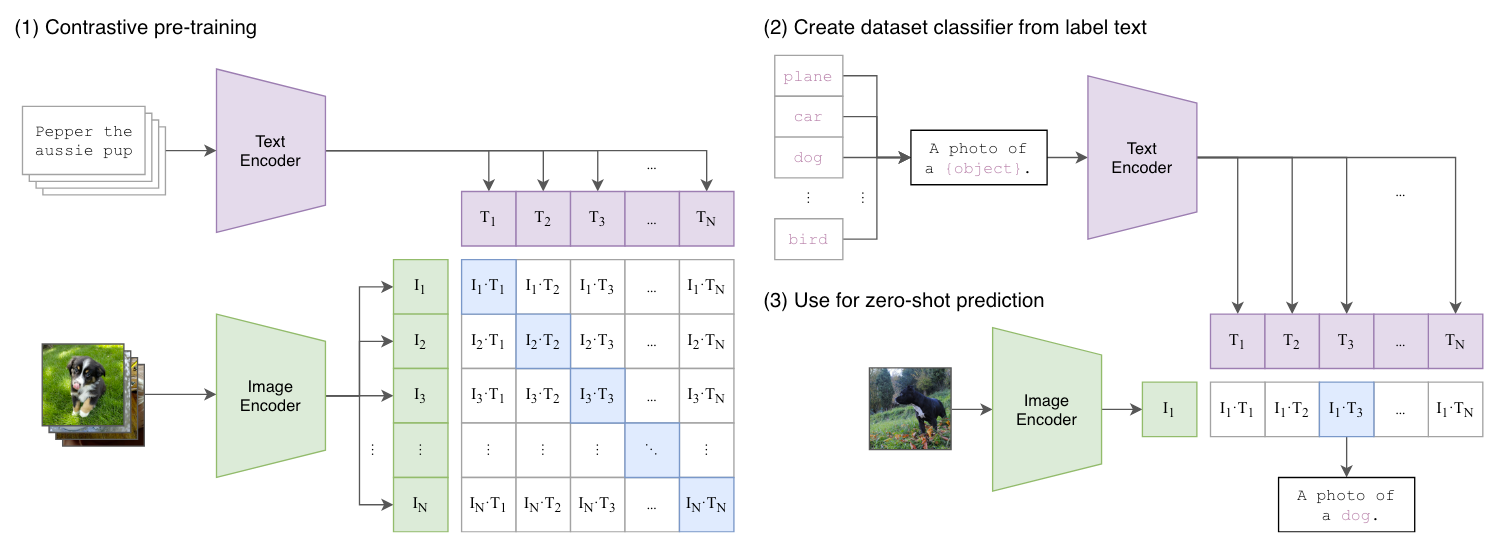# image_encoder - ResNet or Vision Transformer
# text_encoder - CBOW or Text Transformer
# I[n, h, w, c] - minibatch of aligned images
# T[n, l] - minibatch of aligned texts
# W_i[d_i, d_e] - learned proj of image to embed
# W_t[d_t, d_e] - learned proj of text to embed
# t - learned temperature parameter

# extract feature representations of each modality
I_f = image_encoder(I) #[n, d_i]
T_f = text_encoder(T) #[n, d_t]

# joint multimodal embedding [n, d_e]
I_e = l2_normalize(np.dot(I_f, W_i), axis=1)
T_e = l2_normalize(np.dot(T_f, W_t), axis=1)

# scaled pairwise cosine similarities [n, n]
logits = np.dot(I_e, T_e.T) * np.exp(t)

# symmetric loss function
labels = np.arange(n)
loss_i = cross_entropy_loss(logits, labels, axis=0)
loss_t = cross_entropy_loss(logits, labels, axis=1)
loss = (loss_i + loss_t)/2

## ViLT

ViLT的核心思想: 弱Embed重交互: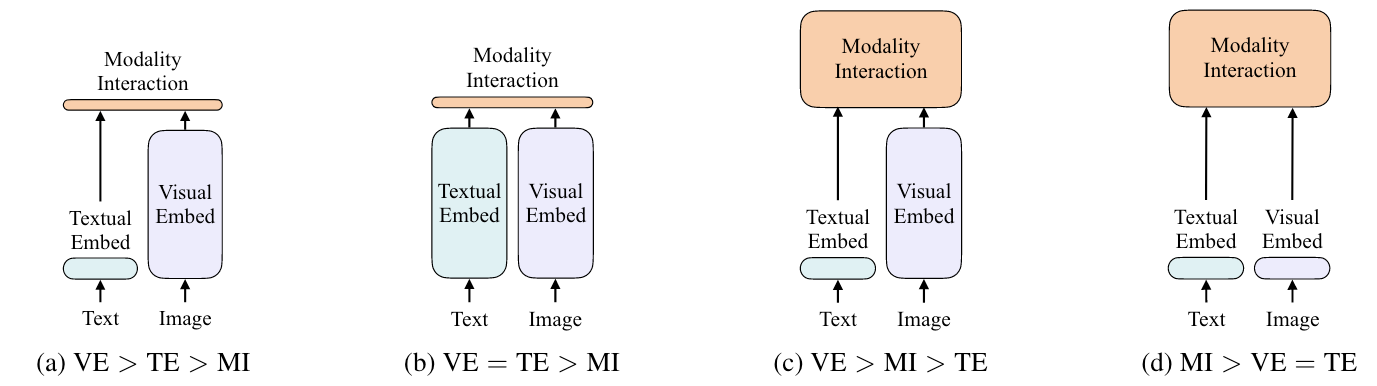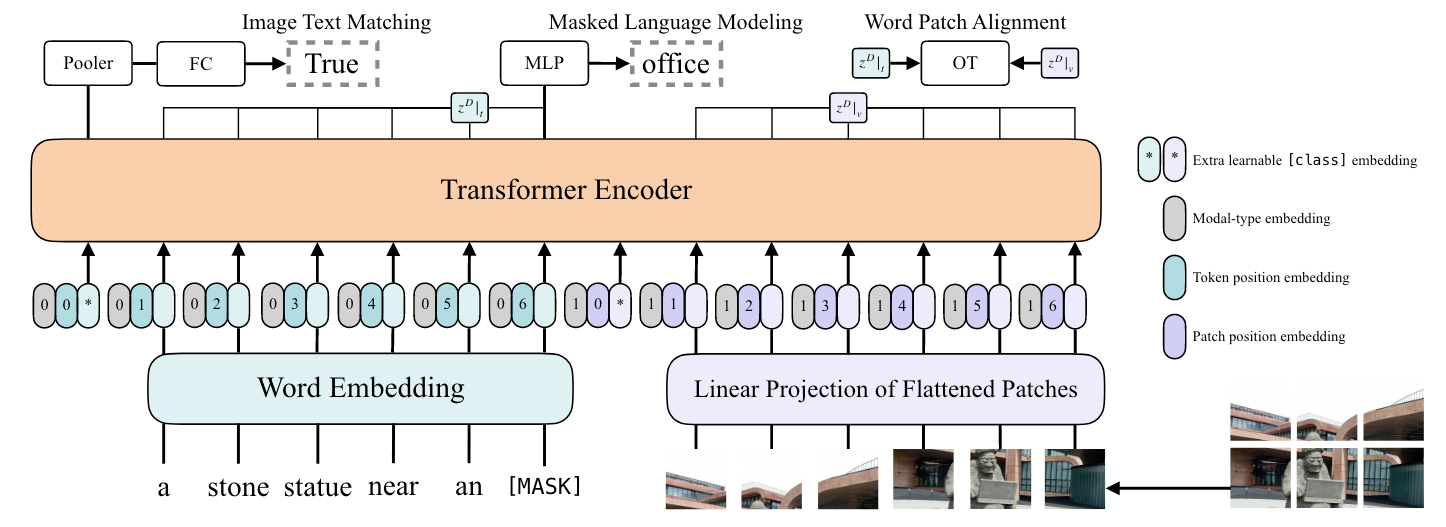## ALBEF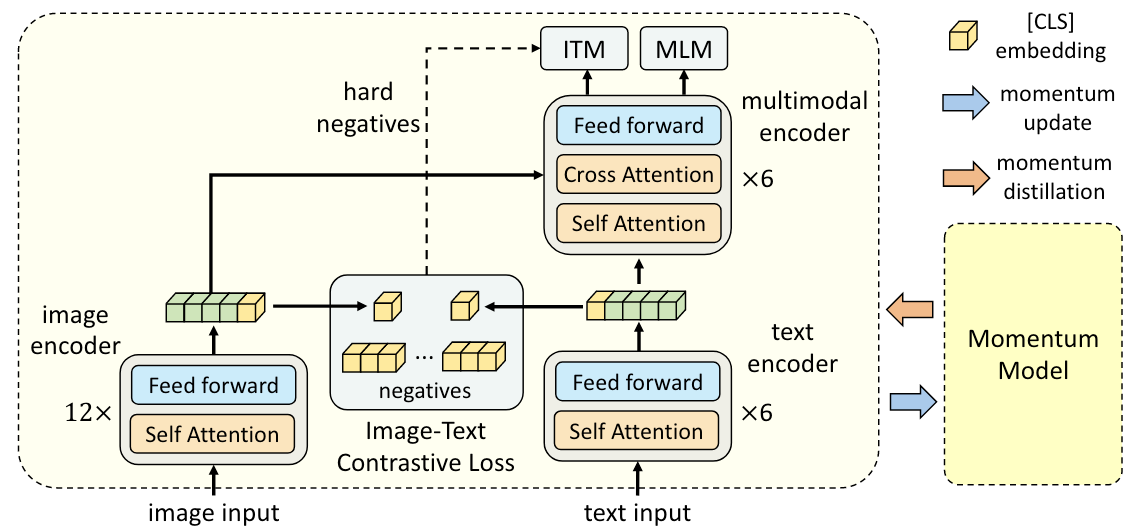ALBEF用了三个Loss: ITC, ITM, MLM, 都是多模态老传统了.

ITC Loss计算如下. 计算主干和Momentum Encoder的图文匹配相似度:

\begin{aligned} s(I, T)=g_v\left(\boldsymbol{v}_{\mathrm{cls}}\right)^{\top} g_w^{\prime}\left(\boldsymbol{w}_{\mathrm{cls}}^{\prime}\right) \\ s(T, I)=g_w\left(\boldsymbol{w}_{\mathrm{cls}}\right)^{\top} g_v^{\prime}\left(\boldsymbol{v}_{\mathrm{cls}}^{\prime}\right) \end{aligned}

$M$ 为图文匹配对数量, 计算InfoNCE:

\begin{aligned} p_m^{\mathrm{i} 2 \mathrm{t}}(I)=\frac{\exp \left(s\left(I, T_m\right) / \tau\right)}{\sum_{m=1}^M \exp \left(s\left(I, T_m\right) / \tau\right)} \\ p_m^{\mathrm{t} 2 \mathrm{i}}(T)=\frac{\exp \left(s\left(T, I_m\right) / \tau\right)}{\sum_{m=1}^M \exp \left(s\left(T, I_m\right) / \tau\right)} \end{aligned}

\begin{aligned} s(I, T)=&g_v\left(\boldsymbol{v}_{\mathrm{cls}}\right)^{\top} g_w^{\prime}\left(\boldsymbol{w}_{\mathrm{cls}}^{\prime}\right) \\ s(T, I)=&g_w\left(\boldsymbol{w}_{\mathrm{cls}}\right)^{\top} g_v^{\prime}\left(\boldsymbol{v}_{\mathrm{cls}}^{\prime}\right) \\ &\Downarrow \\ s^\prime(I, T)=&g_v^\prime\left(\boldsymbol{v}^{\prime}_{\mathrm{cls}}\right)^{\top} g_w^{\prime}\left(\boldsymbol{w}^{\prime}_{\mathrm{cls}}\right) \\ s^\prime(T, I)=&g_w^\prime\left(\boldsymbol{w}^{\prime}_{\mathrm{cls}}\right)^{\top} g_v^{\prime}\left(\boldsymbol{v}^{\prime}_{\mathrm{cls}}\right) \\ \end{aligned}

\begin{aligned} \mathcal{L}_{\mathrm{itc}}=&\frac{1}{2} \mathbb{E}_{(I, T) \sim D}\left[\mathrm{H}\left(\boldsymbol{y}^{\mathrm{i} 2 \mathrm{t}}(I), \boldsymbol{p}^{\mathrm{i} 2 \mathrm{t}}(I)\right)+\mathrm{H}\left(\boldsymbol{y}^{\mathrm{t} 2 \mathrm{i}}(T), \boldsymbol{p}^{\mathrm{t} 2 \mathrm{i}}(T)\right)\right] \\ &\Downarrow \\ \mathcal{L}_{\text {itc }}^{\mathrm{mod}}=&(1-\alpha) \mathcal{L}_{\mathrm{itc}}+\frac{\alpha}{2} \mathbb{E}_{(I, T) \sim D}\left[\mathrm{KL}\left(\boldsymbol{q}^{\mathrm{i} 2 \mathrm{t}}(I) | \boldsymbol{p}^{\mathrm{i} 2 \mathrm{t}}(I)\right)+\mathrm{KL}\left(\boldsymbol{q}^{\mathrm{t} 2 \mathrm{i}}(T) | \boldsymbol{p}^{\mathrm{t} 2 \mathrm{i}}(T)\right)\right] \end{aligned}

MLM任务也做相应的改动:
\begin{aligned} \mathcal{L}_{\mathrm{mlm}}=&\mathbb{E}_{(I, \hat{T}) \sim D} \mathrm{H}\left(\boldsymbol{y}^{\mathrm{msk}}, \boldsymbol{p}^{\mathrm{msk}}(I, \hat{T})\right) \\ &\Downarrow \\ \mathcal{L}_{\mathrm{mlm}}^{\mathrm{mod}}=&(1-\alpha) \mathcal{L}_{\mathrm{mlm}}+\alpha \mathbb{E}_{(I, \hat{T}) \sim D} \mathrm{KL}\left(\boldsymbol{q}^{\mathrm{msk}}(I, \hat{T}) | \boldsymbol{p}^{\mathrm{msk}}(I, \hat{T})\right) \end{aligned}

## BLIP

1. 模型角度: encoder only的模型不擅长文本生成, 而encoder-decoder的模型又不擅长检索.
2. 数据角度: Web端获得的图文匹配对有大量噪声.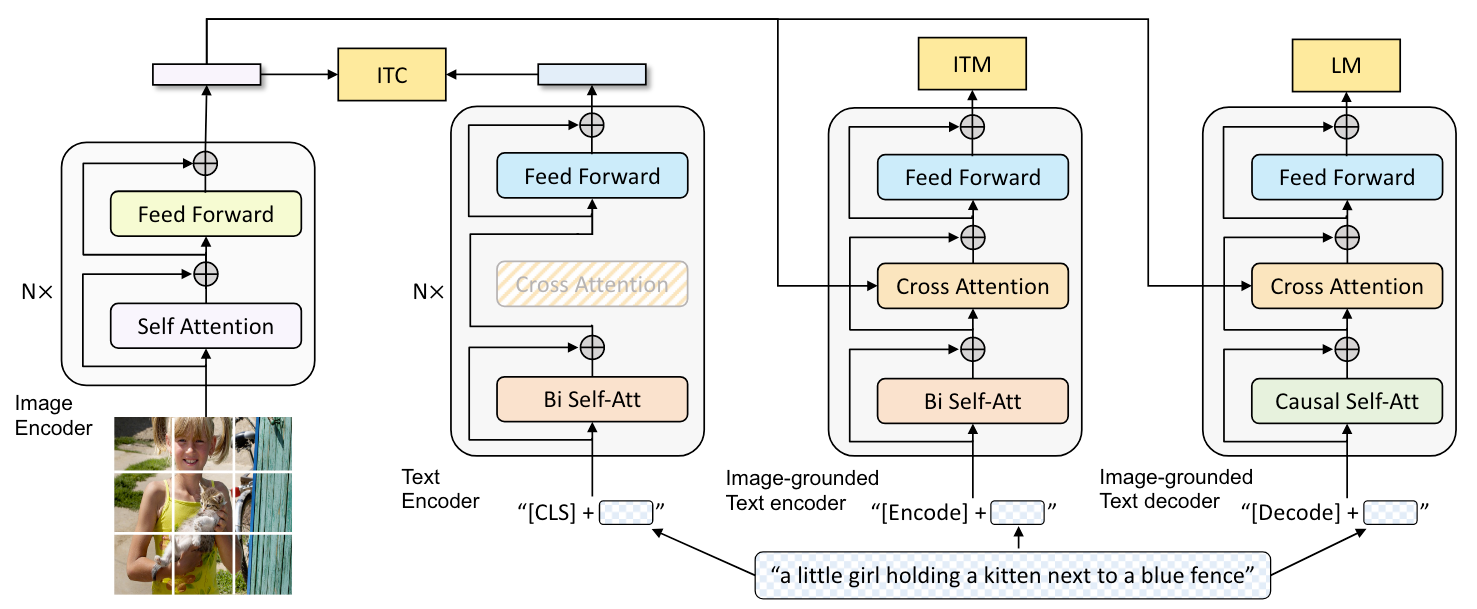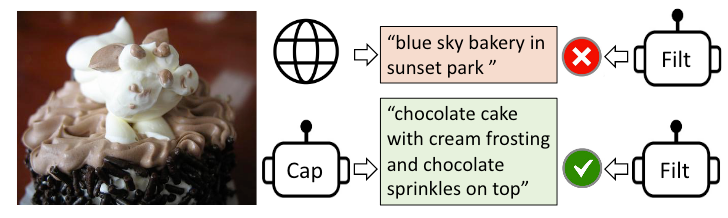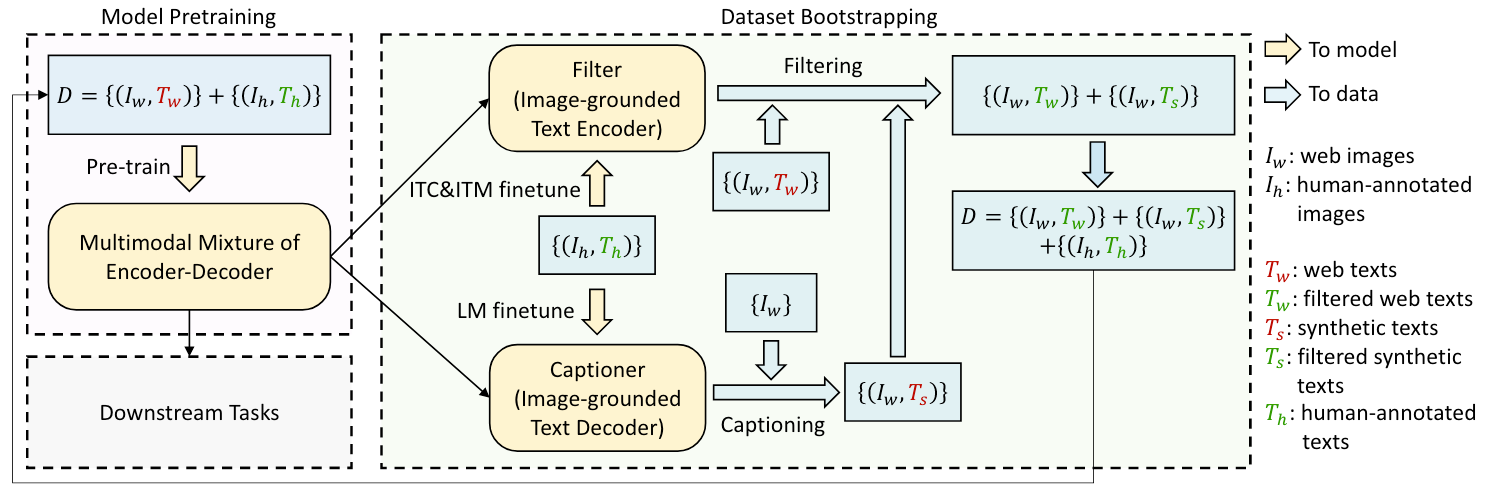BLIP和ALBEF其实思想上非常相似, 从出发点到模型再到左脚踩右脚上天的思想都是一致的.

## VLMo

VLMo对不同模态使用了专家系统(MOE), 大框架如下: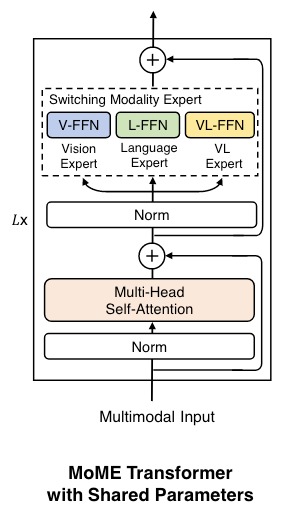SA是可以被共享的, 当VLMo执行不同任务时, 不同的FFN会被启用: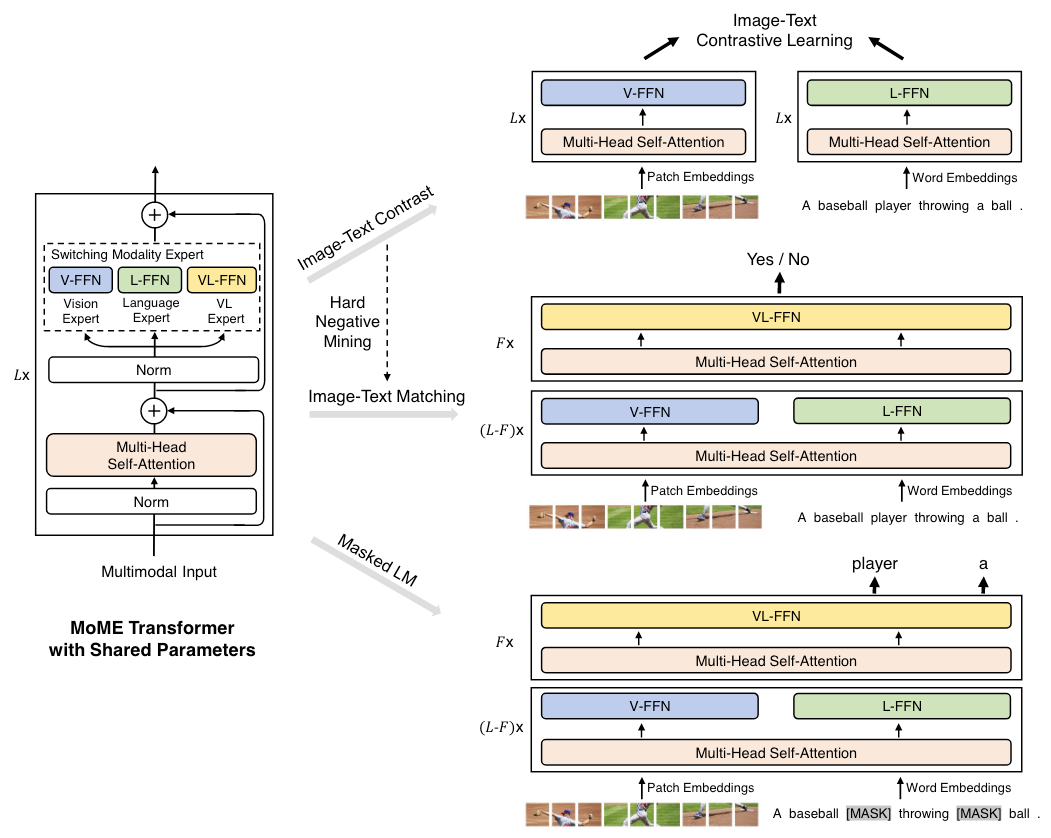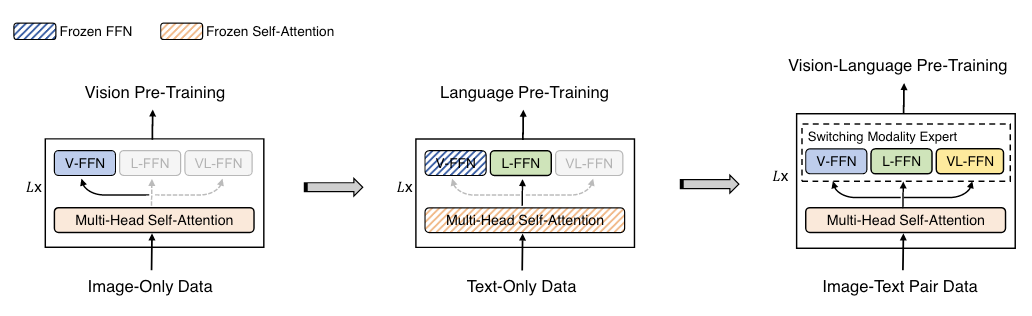## BLIP-2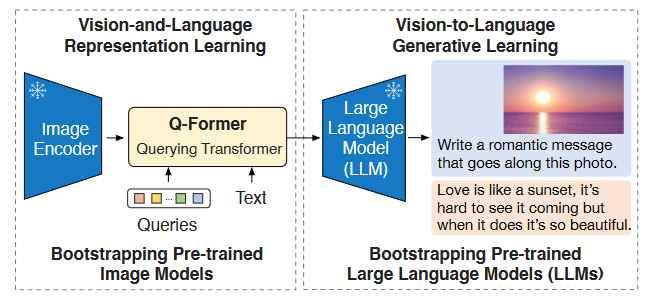• 首阶段: 让Q-Former从Freeze Image Encoder中学习VL表示.
• 次阶段: 从Freeze LLM中学习VL表示.

Q-Former结构和首阶段预训练如下: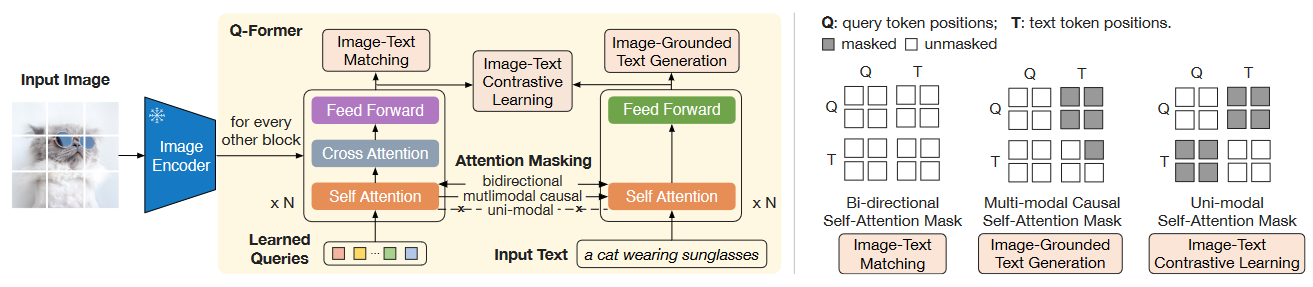Q-Former实际上由双塔的两个Transformer组成, 分别被称为Image Transformer和Text Transformer. 结构上类似于BLIP中的Image-Grounded Text Encoder和Text Encoder.

Image Transformer的SA和Text Transformer的SA参数是共享的(这点和VLMo出奇的一致). Learnable Query从Image Transformer给入, 通过CA来从Frozen Image Encoder中获取视觉信号.

• ITC(Image-Text Contrastive Learning): 虽然说是老生常谈的Loss, 但因为Query经过Trm以后得到的表示有多个, 所以作者计算了多个Query与Text Transformer[CLS]的余弦相似度, 选择相似度最大的作为正样本. 为了避免信息泄露, 在做ITC的时候要保证Q和T之间是互相不可见的(最右侧Mask).
• ITG(Image-grounded Text Generation): 使得Q对T完全可见, T单独用Casual Mask, 然后生成图文匹配的文本段. 这就要求Query必须覆盖Image的全部信息, 且Query抽取出的信息必须是有效的(中间Mask). [CLS]也被换成[DEC].
• ITM(Image-Text Matching): ITM也是常见Loss, Q必须拥有两个模态的信息才能一起判断图文是否匹配, 作者对所有Query都计算ITM Loss, 最后取平均作为Logits, 同时也使用Hard Negative.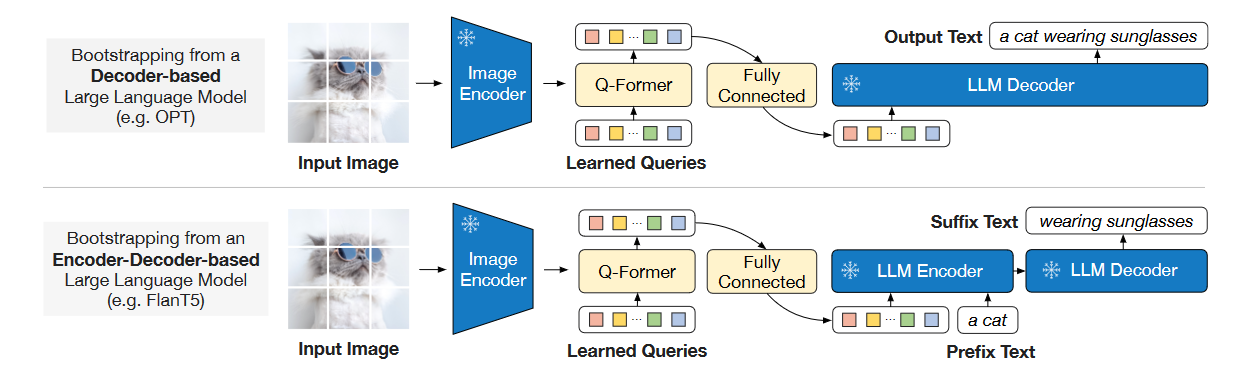本篇Vision & Language Pretrained Model 总结
Vision & Language Pretraining 总结本文只是以总结的形式梳理了近期比较有代表性的VLP模型结构和预训练任务, 推荐有基础后再阅读. UNITER 论文: UNITER: UNiversal Image-T
2023-07-18Large Model并行优化
Large Model并行优化为什么要并行优化?大就是好, 虽然丛2019年人们的认识普遍就是大就是好, 这个概念在当今依然没有被改变, 只是有了更深刻的认识. 所以, 为什么要并行? 虽然大就是好, 模型太大显存吃不消(空间)
2023-06-01
目录